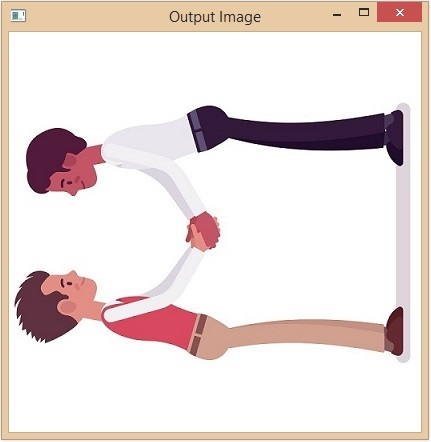# Rotate image without cutting off sides using OpenCV Python

Rotating an image is the most basic operation in image editing. The python OpenCV library provides the methods cv2.getRotationMatrix2D(),cv2.rotate() to do this task very easily.

The cv2.rotate() will rotate the image in 0 or 90 or 180 or 270 angles only where as Cv2.getRotationMatrix2D() will rotate the image to any specified angle. In the article below, we will rotate the image without cropping or cutting off sides using OpenCV Python.

To rotate an image using the cv2.getRotationMatrix2D() method then we need to follow below three steps −

• First, we need to get the centre of rotation.

• Next by using the getRotationMatrix2D() method, we need to create the 2D-rotation matrix.

• Finally, by using the warpAffine() function in OpenCV, we need to apply the affine transformation to the image to correct the geometric distortions or deformations of the image.

## Using Cv2.getRotationMatrix2D() function

The function creates a transformation matrix of the input image array, therefore it will be used for rotating an image. If the value of the angle parameter is positive, then the image gets rotated in the counter-clockwise direction. If you want to rotate the image clockwise, then the angle needs to be negative.

### Syntax

cv2.getRotationMatrix2D(center, angle, scale)


### Parameters

• center: Center of the rotation for the input image.

• angle: The angle of rotation in degrees.

• scale: An isotropic scale factor. Which scales the image up or down according to the value provided.

### Example

Let’s take an example, and rotate the image using the trigonometric functions of the math module.

import cv2
import math

def rotate_image(array, angle):
height, width = array.shape[:2]
image_center = (width / 2, height / 2)

rotation_mat = cv2.getRotationMatrix2D(image_center, angle, 1)

bound_w = int((height * abs(sin)) + (width * abs(cos)))
bound_h = int((height * abs(cos)) + (width * abs(sin)))

rotation_mat[0, 2] += ((bound_w / 2) - image_center)
rotation_mat[1, 2] += ((bound_h / 2) - image_center)

rotated_mat = cv2.warpAffine(array, rotation_mat, (bound_w, bound_h))
return rotated_mat

rotated_image = rotate_image(img, 256)

cv2.imshow('Rotated image', rotated_image)
cv2.waitKey(0)
cv2.destroyAllWindows()


### Input image### Output

The output Rotated image is displayed below.The input image is successfully rotated to the 256 degrees angle.

### Example

In this example, we will rotate an image using cv2.getRotationMatrix2D() and python built in abs() functions.

import cv2

def rotate_image(arr, angle):
height, width = arr.shape[:2]
# get the image centers
image_center = (width/2, height/2)

rotation_arr = cv2.getRotationMatrix2D(image_center, angle, 1)

abs_cos = abs(rotation_arr[0,0])
abs_sin = abs(rotation_arr[0,1])

bound_w = int(height * abs_sin + width * abs_cos)
bound_h = int(height * abs_cos + width * abs_sin)

rotation_arr[0, 2] += bound_w/2 - image_center
rotation_arr[1, 2] += bound_h/2 - image_center

rotated_mat = cv2.warpAffine(arr, rotation_arr, (bound_w, bound_h))
return rotated_arr

rotated_image = rotate_image(img, 197)

cv2.imshow('Original image', img)
cv2.imshow('Rotated image', rotated_image)
cv2.waitKey(0)
cv2.destroyAllWindows()


### Original Image### Rotated ImageThe input image is successfully rotated to the 197degrees angle.

## cv2.rotate()

The cv2.rotate() function rotates an image frame in multiples of 90 degrees(0 or 90 or 180 or 270 angles). The function rotates the image in three different ways using the rotateCode= 0 or 1 or 2 parameters.

### Syntax

cv2.cv.rotate( src, rotateCode[, dst] )


### Parameters

• src: Input image

• rotateCode: It specifies how to rotate the image.

• dst: It is the output image of the same size and depth as the input image.

### Returns

It returns a rotated image.

### Example

In this example, the input image “Fruits.jpg” will be rotated to the 90 degrees anticlockwise direction.

import cv2
import numpy as np

rotated_image = cv2.rotate(img,rotateCode = 2)

cv2.imshow('Original image', img)
cv2.imshow('Rotated image', rotated_image)
cv2.waitKey(0)
cv2.destroyAllWindows()


### Original Image### Rotated Image## Using np.rot90()function

The numpy.rot90() method is used to rotate an array by 90 degrees. If it is sufficient to rotate our input only about 90 degrees rotation, then it is a simple and easier way.

### Example

In this example, we will take an input rectangular image “car.jpg” with 850X315 dimensions.

import cv2
import numpy as np
rotated_image = np.rot90(img)
cv2.imwrite('Rotated image.jpg', rotated_image)
cv2.imshow('InputImage', img)
cv2.waitKey(0)


### Original Image### Rotated ImageThe method rotates the array from the first towards the second axis direction. So that the given image is rotated in an anti-clock wise direction.

Updated on: 30-May-2023

450 Views